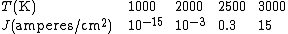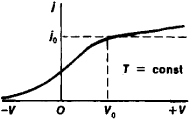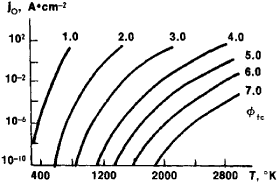thermionic emission

Also found in: Dictionary, Thesaurus, Medical, Legal, Wikipedia.

thermionic emission

(thûrm'īŏn`ĭk), emission of electronselectron,
elementary particle carrying a unit charge of negative electricity. Ordinary electric current is the flow of electrons through a wire conductor (see electricity). The electron is one of the basic constituents of matter.
or ionsion,
atom or group of atoms having a net electric charge. Positive and Negative Electric Charges

A neutral atom or group of atoms becomes an ion by gaining or losing one or more electrons or protons.
by substances that are highly heated, the charged particles being called thermions. The number of thermions emitted increases rapidly as the temperature of the substance rises. The heated material may be in the form of a metal filament or of some compound that coats and is heated by the filament. If the heated body carries a positive or negative charge, the thermions will be of the same charge. At temperatures below red heat (see blackbodyblackbody,
in physics, an ideal black substance that absorbs all and reflects none of the radiant energy falling on it. Lampblack, or powdered carbon, which reflects less than 2% of the radiation falling on it, crudely approximates an ideal blackbody; a material consisting of a
), thermionic emission from uncharged bodies is chiefly positive; at higher temperatures it is negative. The effect was discovered by Thomas A. Edison in 1883 when he was working on filaments for the electric light. Thermionic emission's most important practical application in electronics is in the electron tubeelectron tube,
device consisting of a sealed enclosure in which electrons flow between electrodes separated either by a vacuum (in a vacuum tube) or by an ionized gas at low pressure (in a gas tube).
, since it is the mechanism by which electrons are emitted from the cathode.

Thermionic emission

The emission of electrons into vacuum by a heated electronic conductor. In its broadest meaning, thermionic emission includes the emission of ions, but this process is quite different from that normally understood by the term. Thermionic emitters are used as cathodes in electron tubes and hence are of great technical and scientific importance. Although in principle all conductors are thermionic emitters, only a few materials satisfy the requirements set by practical applications. Of the metals, tungsten is an important practical thermionic emitter; in most electron tubes, however, the oxide-coated cathode is used to great advantage.

The thermionic emission of a material may be measured by using the material as the cathode in a vacuum tube and collecting the emitted electrons on a positive anode. If the anode is sufficiently positive relative to the cathode, space charge (a concentration of electrons near the cathode) can be avoided and all electrons emitted can be collected; the saturation thermionic current is then measured. See Schottky effect

The emission current density J increases rapidly with increasing temperature; this is illustrated by the following approximate values for tungsten:The temperature dependence of J is given by the Richards (or Dushman-Richardson) equation below.Here A is a constant, k is Boltzmann's constant (=1.38 × 10-23 joule/ degree), and &phgr; is the work function of the emitter. The work function has the dimensions of energy and is a few electronvolts for thermionic emitters. See Work function (electronics)

McGraw-Hill Concise Encyclopedia of Physics. © 2002 by The McGraw-Hill Companies, Inc.
The following article is from The Great Soviet Encyclopedia (1979). It might be outdated or ideologically biased.

Thermionic Emission

(or Edison effect, Richardson effect), the emission of electrons by hot solids or, less often, liquids into a vacuum or various media. In its broadest sense, “thermionic emission” includes the emission of ions. The present article, however, deals only with the case of electron emission.Figure 1. Current-voltage characteristic, that is, the dependence of the thermionic current density (i) on the potential difference (V) applied between the electron emitter and electron collector

The phenomenon was first investigated by O. W. Richardson in 1900 and 1901. Thermionic emission can be regarded as the evaporation of electrons owing to their thermal excitation. To leave the emitting body, the electrons must overcome the potential barrier at the boundary of the body. When the body is at low temperatures, the number of electrons with enough energy to overcome the barrier is small. As the temperature increases, the number of such electrons rises, and the thermionic emission increases (see).

The principal quantity characterizing bodies with respect to thermionic emission is the saturation thermionic current density j0 at a specified temperature (Figure 1). For thermionic emission by uniform (with respect to the work function) emitters into a vacuum in the absence of external electric fields, the quantity j0 is given by the Richardson-Dushman equation

(1) j0 = AT2(1 – r̄) exp [– eϕ (T)/KT]

Here, A is a constant of the emitter, T is the temperature of the emitter in °K, r̄ is the average coefficient of reflection from the potential barrier at the boundary of the emitter for thermoelectrons with different energies, eϕ is the work function, and k is the Boltzmann constant. For metals, in the Sommerfeld free-electron model, A = A0 = 4πek2m/h3 = 120.4 amperes/°K2cm2, where e is the charge of the electron, m is the mass of the electron, and h is Planck’s constant. The electrons emitted have a Maxwellian distribution of initial velocities that corresponds to the temperature of the emitter.

During thermionic emission into a vacuum, the electrons form at the surface of the emitter a space charge whose electric field retards electrons with low initial velocities. An electric field that compensates for the field of the space charge is therefore created to obtain a saturation current between the electron emitter (the cathode) and the electron collector (the anode). The current-voltage characteristic of a vacuum diode with a thermionic cathode is shown in Figure 1. The saturation current density j0 is reached at a potential difference V0 whose value is determined by the Langmuir formula. When V < V0, the current is limited by the field of the space charge at the surface of the emitter. The slight increase in j when V > V0 is due to the Schottky effect. Figure 1 shows that a thermionic current can also flow in the absence of applied electromotive forces. This fact indicates the possibility of creating vacuum thermionic converters of thermal energy into electrical energy. In applied electric fields with a strength E ≥ 106–107 volts/cm, field, or cold, emission is added to thermionic emission.

For metals and intrinsic semiconductors, ϕ may be assumed linearly dependent on T in narrow temperature ranges ΔT containing a chosen T0: ϕ(T) = ϕ(T0) + α(TT0), where α is the rate of change of ϕ with temperature in the temperature range ΔT in question. In this case, equation (1) may be written in the form

(2) j0 = ART2 exp (– eϕR/KT)

where AR = A(1 – r̄) exp (– eα/k) is called the Richardson constant of the emitter, which is uniform with respect to the work function; eϕR = ϕ(T0) – αT0;eϕ0 is called the Richardson work function. In the temperature range from T = 0 to T = T0, α does not retain a constant value; consequently, the Richardson work function differs from the true work function of the electrons at a temperature T = 0°K. The quantities AR and eϕR are found from straight-line graphs of the function In (j0/T2) = f(1/T); such graphs are called Richardson plots. In impurity semiconductors the function ϕ(T) is more complicated, and the equation for j0 differs from equation (2).

The quantities A and r̄ in equation (1), which are unknown for most emitters, depend not only on the material of the emitter but also on the state of its surface; they are determined experimentally. To eliminate these quantities, the equation is reduced to the form

(3) j = A0T2 exp [– eϕtc(T)/kT]

The work function eϕtc(T) differs little in value from the true work function of the emitter eϕ(T) and is easily determined from the measured values of j0 and T; it represents the work function for the total emission current. The quantity eϕtc uniquely characterizes the thermionic properties of an emitter; knowledge of this quantity permits j0(T) to be found (Figure 2).Figure 2. Saturation thermionic current density at different temperatures and for different work functions eϕ, as determined from the total thermionic current

The faces of ideal single crystals, both clean faces and those coated with homogeneous films of another substance, are uniform emitters with respect to ϕ. The majority of the emitters used in practice are not uniform, but consist of patches with different ϕ. Examples are polycrystalline emitters, emitters with structural defects, and two-phase film emitters. The contact potential differences between patches lead to the appearance of local patch fields over the emitting surface. These fields create additional barriers to the emission of electrons from the patches where the work function is less than the average for the surface and result in the anomalous Schottky effect. To describe the thermionic emission of nonuniform emitters, averaged quantities characterizing the emission are introduced into equation (1).

To obtain high-density currents that are constant over time, emitters with small ϕ and high heats of vaporization for the material are required. In many cases special requirements, such as chemical passivity and corrosion resistance, are imposed on thermionic emitters. A high thermionic capacity is exhibited by the so-called effective cathodes, such as barium oxide cathodes, thorium oxide cathodes, and cathodes made with hexaborides of alkaline-earth and rare-earth metals. Good thermionic emission is also exhibited by some metallic film cathodes, such as refractory-metal cathodes coated with a film of an alkali, alkaline-earth, or rare-earth metal.

Thermionic emission underlies the operation of many electrical vacuum and gas discharge instruments and devices.

REFERENCES

Reimann, A. L. Termoionnaia emissiia. Moscow-Leningrad, 1940. (Translated from English.)
Gaponov, V. I. Elektronika, vol. 1. Moscow, 1960.
Dobretsov, L. N., and M. V. Gomoiunova. Emissionnaia elektronika. Moscow, 1966.
Knoll, M., and J. Eüchmeier. Tekhnicheskaia elektronika, vol. 1. Moscow, 1971. (Translated from German.)
Herring, C., and M. Nichols. Termoelektronnaia emissiia. Moscow, 1950. (Translated from English.)
Zandberg, E. Ia., and N. I. Ionov. Poverkhnostnaia ionizatsiia. Moscow, 1969.
Fomenko, V. S. Emissionnye svoistva materialov. Kiev, 1970.

E. IA. ZANDBERG

thermionic emission

[‚thər·mē′än·ik i′mish·ən]
(electronics)
The outflow of electrons into vacuum from a heated electric conductor. Also known as Edison effect; Richardson effect.
More broadly, the liberation of electrons or ions from a substance as a result of heat.
McGraw-Hill Dictionary of Scientific & Technical Terms, 6E, Copyright © 2003 by The McGraw-Hill Companies, Inc.
References in periodicals archive ?
Schottky or Thermionic Emission. Schottky emission is a conduction mechanism that if the electrons can obtain enough energy provided by thermal activation, the electrons in the metal will overcome the energy barrier at the metal-dielectric interface to go to the dielectric.
At such a low temperature, the thermionic emission is suppressed and the tunneling current is dominant.
To identify electron effective mass and barrier height, it is useful to measure the thermionic emission current at high temperature and the tunneling current at low temperature.
In the discussion of thermionic emission over the insulator-semiconductor barrier on [I.sub.MIS], we obtain 
At this point, the number of holes (minority carriers) at the surface is larger than the number of the electrons (majority carrier), and thermionic emission of electrons is recombined by holes.
The ideality factor ([eta]), a dimensionless quantity, is a measure of the conformity of the diode behavior to pure thermionic emission. It is introduced to account for the deviation from the thermionic emission diffusion theory.
The forward I-V characteristics of NPANI/PSi SB diode, according to thermionic emission theory , are expressed by (1).

Site: Follow: Share:
Open / Close